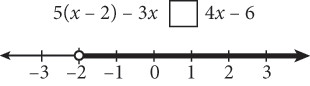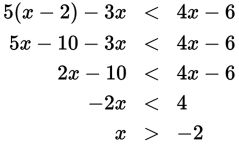# SAT Math Multiple Choice Question 553: Answer and Explanation

### Test Information

Question: 553

13.Which symbol correctly completes the inequality whose solution is shown above?

• A. <
• B. >
• C. ≤
• D. ≥

Explanation:

A

Difficulty: Medium

Category: Heart of Algebra / Inequalities

Strategic Advice: Apply logic to this question first, and then algebra. The dot at the beginning of the shaded portion is an open dot, so –2 is not included in the solution set of the inequality. This means you can eliminate C and D because those symbols would include the endpoint. Don't immediately choose B just because the arrow is pointing to the right, which typically indicates greater than. When dealing with an inequality, if you multiply or divide by a negative number, you must flip the symbol, so the answer is not necessarily what you might think.

Getting to the Answer: Because you were able to eliminate two of the choices, the quickest approach is to pick one of the remaining symbols, plug it in, and see if it works. If it does, choose that answer. If it doesn't, then it must be the other symbol. Try (A):The resulting inequality, x > –2, means all the values on the number line greater than (or to the right of) –2, so the initial inequality symbol must have been <. Choice (A) is correct.